### IMO Shortlist 1996 problem G1

Kvaliteta:
Avg: 5,0
Težina:
Avg: 5,0
Let$ABC$ be a triangle, and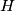$H$ its orthocenter. Let$P$ be a point on the circumcircle of triangle$ABC$ (distinct from the vertices$A$,$B$,$C$), and let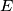$E$ be the foot of the altitude of triangle$ABC$ from the vertex$B$. Let the parallel to the line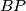$BP$ through the point$A$ meet the parallel to the line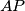$AP$ through the point$B$ at a point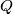$Q$. Let the parallel to the line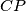$CP$ through the point$A$ meet the parallel to the line$AP$ through the point$C$ at a point$R$. The lines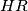$HR$ and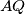$AQ$ intersect at some point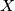$X$. Prove that the lines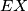$EX$ and$AP$ are parallel.
Izvor: Međunarodna matematička olimpijada, shortlist 1996